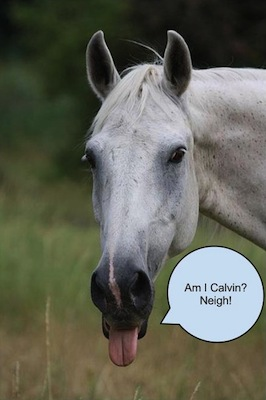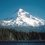# You are inductively CalvinSee the Blog for the full story.

Are you convinced that induction has proven your name to be Calvin?

Just how powerful is Induction? I'd use it to show that your name is Calvin.

Claim: In a group of $n$ people, everyone has the same name.

Proof: Let's show that condition (1) holds. In a group of $n=1$ people, all of them have the same name.

Let's show that condition (2) holds. Since the positive integer $k$ is in the set, this means that in a group of any $k$ people, all of them have the same name. So let's consider a group of $k+1$ people. Since the first $k$ people have the same name, and the last $k$ people also have the same name, it means that all $k+1$ of them must have the same name.

Thus, by Mathematical Induction, the set $S$ includes all positive integers, so in a group of $n$ people, everyone has the same name.

In particular, since my name is Calvin, and you are in the set of all $N$ people on Earth, it follows that your name is also Calvin.

The above is adapted from Polya's proof that "No horse is of a different color".Note by Peter Taylor
7 years, 5 months ago

This discussion board is a place to discuss our Daily Challenges and the math and science related to those challenges. Explanations are more than just a solution — they should explain the steps and thinking strategies that you used to obtain the solution. Comments should further the discussion of math and science.

When posting on Brilliant:

• Use the emojis to react to an explanation, whether you're congratulating a job well done , or just really confused .
• Ask specific questions about the challenge or the steps in somebody's explanation. Well-posed questions can add a lot to the discussion, but posting "I don't understand!" doesn't help anyone.
• Try to contribute something new to the discussion, whether it is an extension, generalization or other idea related to the challenge.

MarkdownAppears as
*italics* or _italics_ italics
**bold** or __bold__ bold
- bulleted- list
• bulleted
• list
1. numbered2. list
1. numbered
2. list
Note: you must add a full line of space before and after lists for them to show up correctly
paragraph 1paragraph 2

paragraph 1

paragraph 2

[example link](https://brilliant.org)example link
> This is a quote
This is a quote
    # I indented these lines
# 4 spaces, and now they show
# up as a code block.

print "hello world"
# I indented these lines
# 4 spaces, and now they show
# up as a code block.

print "hello world"
MathAppears as
Remember to wrap math in $$ ... $$ or $ ... $ to ensure proper formatting.
2 \times 3 $2 \times 3$
2^{34} $2^{34}$
a_{i-1} $a_{i-1}$
\frac{2}{3} $\frac{2}{3}$
\sqrt{2} $\sqrt{2}$
\sum_{i=1}^3 $\sum_{i=1}^3$
\sin \theta $\sin \theta$
\boxed{123} $\boxed{123}$

Sort by:

Fails at $k = 1$, since the first 1 person does not 'intersect' with the last 1 person if there are 2.

- 7 years, 5 months ago

I don't get it... neither the proof nor the anti-proof.

What does the proof by induction, which deals with integer values, have to do with categorical variables such as a name? One has a magnitude the other one doesn't... They're not in the same domain.

- 5 years, 9 months ago

`Hmm...Calvin is pretty famous :D

- 7 years, 5 months ago

Yes there is a gap in $k=1$. I think this post is meant to remind other Brilliant users about some tricky spots when you are writing a solution.

- 7 years, 5 months ago

let k=1; Because the first 1 person has the same name, and the last one person has the same name, then both people have the same name? Obvious bogus :P

- 7 years, 5 months ago

Spoiler: To spoil the fun, here's the reason why its false. (Don't see until you are totally sure you cannot make out the goof-up)

Peace.

Faustus

- 7 years, 5 months ago

This was discussed in Mathematical Induction II-B.

- 7 years, 5 months ago

Say two students are $A$ and $B$.Then according to above proof,$A$'s name is $A$ ,and $B$ at the same time.The same applies to $B$,so we have a contradiction.So,if the proof is true,wouldn't everybody's name be Calvin/Zi Song/Abhishek/......... at the same time? :D

- 7 years, 5 months ago

I think this is what we want to prove.

- 7 years, 5 months ago

I agree.Anyway that's just a joke. :D

- 7 years, 5 months ago

the proof is wrong at the assumption of p(k) , according to this assumption , in a group of any k people, all of them have the same name. lets have two sets of people having k people each , A= {a1, a2, ..........ak}, B={b1 , b2............bk}, by our assumption of p(k), every one in set A has same name , let it be Calvin, and everyone in set B also has same name which may or may not be calvin , suppose let it be abcd, now take a new set C={a1, a2,......ar, b1, b2, ......bq}, such that r+q=k, so the elements of this set has same name so abcd must be same as Calvin, this happens for every set of k people, so all the people in the universal set must have same name(earth), but then we don 't need p(k+1), to prove the result , so this is not proved inductively. as in induction we must have 1) p(1) is true 2) assume p(k) is true and using this prove p(k+1),

but here our assumption itself solves the proof so it cannot be done inductively

- 7 years, 5 months ago

The mass argument (the 2nd one) does not make sense to me ; i there a typo in it?

- 6 years, 7 months ago